Systems of Linear Equations:
(page 5 of 7)

Sections: Definitions, Solving by graphing, Substitition, Elimination/addition, Gaussian elimination.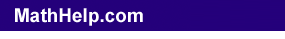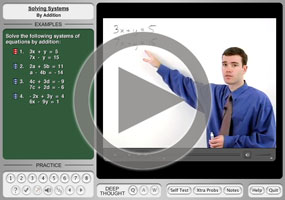The addition method of solving systems of equations is also called the method of elimination. This method is similar to the method you probably learned for solving simple equations.

If you had the equation "x + 6 = 11", you would write "–6" under either side of the equation, and then you'd "add down" to get "x = 5" as the solution.

x + 6 = 11
–6    –6
x       =   5

You'll do something similar with the addition method.

• Solve the following system using addition.
• 2x + y = 9
3
xy = 16

Note that, if I add down, the y's will cancel out. So I'll draw an "equals" bar under the system, and add down:

2x + y = 9
3xy = 16
5x      = 25

Now I can divide through to solve for x = 5, and then back-solve, using either of the original equations, to find the value of y. The first equation has smaller numbers, so I'll back-solve in that one:

2(5) + y = 9
10 + y = 9
y = –1

Then the solution is (x, y) = (5, –1).

It doesn't matter which equation you use for the backsolving; you'll get the same answer either way. If I'd used the second equation, I'd have gotten:

3(5) – y = 16
15 – y = 16
–y = 1
y = –1

...which is the same result as before.

• Solve the following system using addition.
• x – 2y = –9
x + 3y = 16

Note that the x-terms would cancel out if only they'd had opposite signs. I can create this cancellation by multiplying either one of the equations by –1, and then adding down as usual. It doesn't matter which equation I choose, as long as I am careful to multiply the –1 through the entire equation. (That means both sides of the "equals" sign!)

I'll multiply the second equation.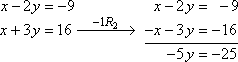The "–1R2" notation over the arrow indicates that I multiplied row 2 by –1. Now I can solve the equation "–5y = –25" to get y = 5. Back-solving in the first equation, I get:

x – 2(5) = –9
x – 10 = –9
x = 1

Then the solution is (x, y) = (1, 5).

A very common temptation is to write the solution in the form "(first number I found, second number I found)". Sometimes, though, as in this case, you find the y-value first and then the x-value second, and of course in points the x-value comes first. So just be careful to write the coordinates for your solutions correctly. Copyright © Elizabeth Stapel 2003-2011 All Rights Reserved

Content Continues Below

• Solve the following system using addition.
• 2x –   y =     9
3
x + 4y = –14

Nothing cancels here, but I can multiply to create a cancellation. I can multiply the first equation by 4, and this will set up the y-terms to cancel.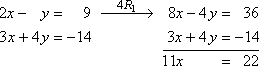Solving this, I get that x = 2. I'll use the first equation for backsolving, because the coefficients are smaller.

2(2) – y = 9
4 – y = 9
y = 5
y = –5

The solution is (x, y) = (2, –5).

• Solve the following system using addition.
• 4x – 3y = 25
–3
x + 8y = 10

Hmm... nothing cancels. But I can multiply to create a cancellation. In this case, neither variable is the obvious choice for cancellation. I can multiply to convert the x-terms to 12x's or the y-terms to 24y's. Since I'm lazy and 12 is smaller than 24, I'll multiply to cancel the x-terms. (I would get the same answer in the end if I set up the y-terms to cancel. It's not that how I'm doing it is "the right way"; it was just my choice. You could make a different choice, and that would be just as correct.)

I will multiply the first row by 3 and the second row by 4; then I'll add down and solve.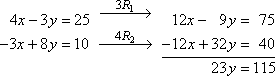Solving, I get that y = 5. Neither equation looks particularly better than the other for back-solving, so I'll flip a coin and use the first equation.

4x – 3(5) = 25
4x – 15 = 25
4x = 40
x = 10

Remembering to put the x-coordinate first in the solution, I get:

(x, y) = (10, 5)

Usually when you are solving "by addition", you will need to create the cancellation. Warning: The most common mistake is to forget to multiply all the way through the equation, multiplying on both sides of the "equals" sign. Be careful of this.

• Solve the following using addition.
• 12x –  13y =   2
–6
x + 6.5y = –2

I think I'll multiply the second equation by 2; this will at least get rid of the decimal place.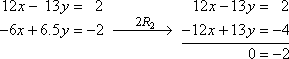Oops! This result isn't true! So this is an inconsistent system (two parallel lines) with no solution (with no intersection point).

no solution

• Solve the following using addition.
• 12x – 3y = 6
4
x –   y = 2

I think it'll be simplest to cancel off the y-terms, so I'll multiply the second row by –3.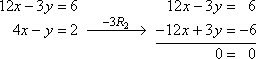Well, yes, but...? I already knew that zero equals zero. So this is a dependent system, and, solving for "y =", the solution is:

y = 4x – 2

(Your text may format the answer as "(s, 4s – 2)", or something like that.)

Remember the difference: a nonsense answer (like "0 = –2" in the previous problem) means an inconsistent system with no solution; a useless answer (like "0 = 0" above) means a dependent system where the whole line is the solution.

Some books use only "x" and "y" for their variables, but many use additional variables. When you write the solution for an x,y-point, you know that the x-coordinate goes first and the y-coordinate goes second. When you are dealing with other variables, assume (unless explicitly told otherwise) that those variables are written in alphabetical order. For instance, if the variables in a given system are a and b, the solution point would be (a, b); it would not be (b, a). Unless otherwise specified, the variables are written in alphabetical order.

<< Previous  Top  |  1 | 2 | 3 | 4 | 5 | 6 | 7  |  Return to Index  Next >>

 Cite this article as: Stapel, Elizabeth. "Systems of Linear Equations: Solving by Addition / Elimination." Purplemath. Available from     https://www.purplemath.com/modules/systlin5.htm. Accessed [Date] [Month] 2016

MathHelp.com Courses

Purplemath:

Study Skills Survey